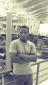# Spherical Trigonometry: Unknown vertex angle and it's opposite side

4 posts / 0 new
123Meisnoob
Spherical Trigonometry: Unknown vertex angle and it's opposite side

An isosceles spherical triangle has angle A=B = 54° , and side b = 82° find the measure of the third angle

Jhun Vert$\dfrac{\sin a}{\sin A} = \dfrac{\sin b}{\sin B}$

$a = \checkmark$

With side "a" already known, you may use Napier's analogy or you may use simultaneous equations of cosine law for sides and cosine law for angles.

By Napier's analogy:

$\dfrac{\sin \frac{1}{2}(A - B)}{\sin \frac{1}{2}(A + B)} = \dfrac{\tan \frac{1}{2}(a - b)}{\tan \frac{1}{2}C}$

$C = \checkmark$       answer

By simultaneous equations

Cosine Law for Angles
$\cos C = -\cos A ~ \cos B + \sin A ~ \sin B ~ \cos c$

$\cos C - \sin A ~ \sin B ~ \cos c = -\cos A ~ \cos B$

$x - (\sin A ~ \sin B)y = -\cos A ~ \cos B$   ←   Equation (1)

Cosine Law for Sides
$\cos c = \cos a ~ \cos b + \sin a ~ \sin b ~ \cos C$

$\sin a ~ \sin b ~ \cos C - \cos c = -\cos a ~ \cos b$

$(\sin a ~ \sin b)x - y = -\cos a ~ \cos b$   ←   Equation (2)

Solve for x
$\cos C = x$

$C = \checkmark$       answer

Boaz KatatumbaGreat from you sir. I can really learn a lot from here. Mechanical engineering student

Jhun VertWelcome to MATHalino.com sir Boaz. Enjoy your stay.

• Mathematics inside the configured delimiters is rendered by MathJax. The default math delimiters are $$...$$ and $...$ for displayed mathematics, and $...$ and $...$ for in-line mathematics.